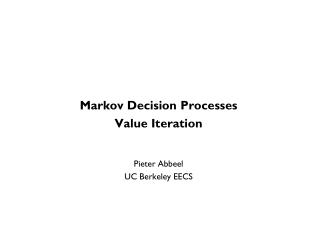Download PresentationMarkov Decision Processes Value Iteration Pieter Abbeel UC Berkeley EECS

# Markov Decision Processes Value Iteration Pieter Abbeel UC Berkeley EECS - PowerPoint PPT Presentation

Markov Decision Processes Value Iteration Pieter Abbeel UC Berkeley EECS. TexPoint fonts used in EMF. Read the TexPoint manual before you delete this box.: A A A A A A A A A A A. Markov Decision Process. [Drawing from Sutton and Barto , Reinforcement Learning: An Introduction, 1998].I am the owner, or an agent authorized to act on behalf of the owner, of the copyrighted work described.
Download Presentation## Markov Decision Processes Value Iteration Pieter Abbeel UC Berkeley EECS

Download Policy: Content on the Website is provided to you AS IS for your information and personal use and may not be sold / licensed / shared on other websites without getting consent from its author.While downloading, if for some reason you are not able to download a presentation, the publisher may have deleted the file from their server.

- - - - - - - - - - - - - - - - - - - - - - - - - - E N D - - - - - - - - - - - - - - - - - - - - - - - - - -
Presentation Transcript
1. Markov Decision Processes Value Iteration Pieter Abbeel UC Berkeley EECS TexPoint fonts used in EMF. Read the TexPoint manual before you delete this box.: AAAAAAAAAAA

2. Markov Decision Process [Drawing from Sutton and Barto, Reinforcement Learning: An Introduction, 1998] Assumption: agent gets to observe the state

3. Markov Decision Process (S, A, T, R, H) • Given • S: set of states • A: set of actions • T: S x A x S x {0,1,…,H}  [0,1], Tt(s,a,s’) = P(st+1 = s’ | st = s, at =a) • R: S x A x S x {0, 1, …, H}  < Rt(s,a,s’) = reward for (st+1 = s’, st= s, at =a) • H: horizon over which the agent will act • Goal: • Find ¼ : S x {0, 1, …, H}  A that maximizes expected sum of rewards, i.e.,

4. Examples MDP (S, A, T, R, H), goal: • Cleaning robot • Walking robot • Pole balancing • Games: tetris, backgammon • Server management • Shortest path problems • Model for animals, people

5. Canonical Example: Grid World • The agent lives in a grid • Walls block the agent’s path • The agent’s actions do not always go as planned: • 80% of the time, the action North takes the agent North (if there is no wall there) • 10% of the time, North takes the agent West; 10% East • If there is a wall in the direction the agent would have been taken, the agent stays put • Big rewards come at the end

6. Grid Futures Deterministic Grid World Stochastic Grid World E N S W E N S W ?

7. Solving MDPs • In an MDP, we want an optimal policy *: S x 0:H → A • A policy  gives an action for each state for each time • An optimal policy maximizes expected sum of rewards • Contrast: In deterministic, want an optimal plan, or sequence of actions, from start to a goal t=5=H t=4 t=3 t=2 t=1 t=0

8. Value Iteration • Idea: = the expected sum of rewards accumulated when starting from state s and acting optimally for a horizon of i steps • Algorithm: • Start with for all s. • For i=1, … , H Given Vi*, calculate for all states s 2 S: • This is called a value update or Bellman update/back-up

9. Example

10. Example: Value Iteration • Information propagates outward from terminal states and eventually all states have correct value estimates V2 V3

11. Practice: Computing Actions • Which action should we chose from state s: • Given optimal values V*? • = greedy action with respect to V* • = action choice with one step lookaheadw.r.t. V*

12. Today and forthcoming lectures • Optimal control: provides general computational approach to tackle control problems. • Dynamic programming / Value iteration • Discrete state spaces (DONE!) • Discretization of continuous state spaces • Linear systems • LQR • Extensions to nonlinear settings: • Local linearization • Differential dynamic programming • Optimal Control through Nonlinear Optimization • Open-loop • Model Predictive Control • Examples: